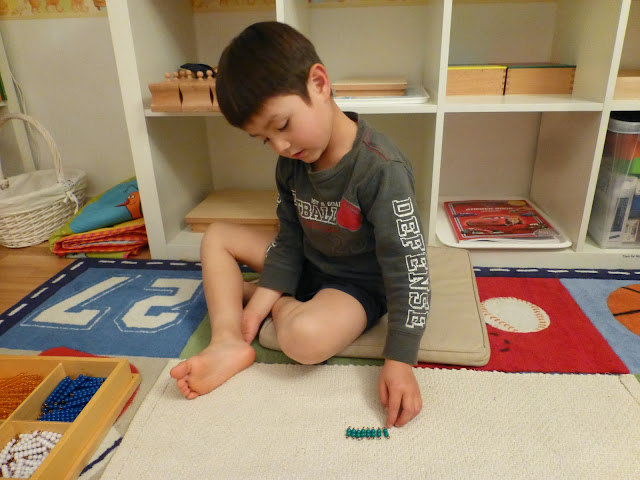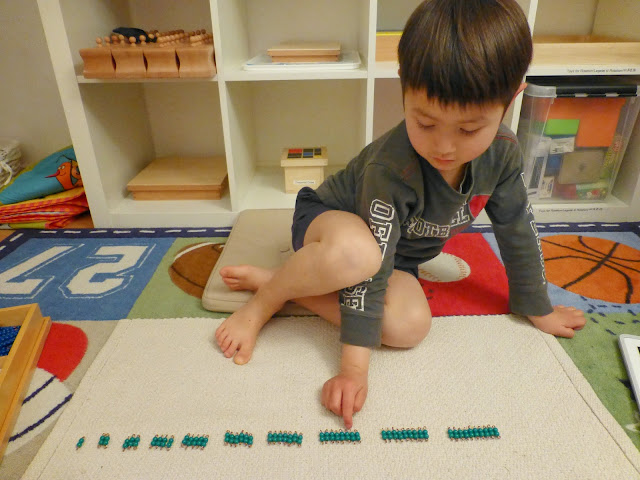## Monday, 12 May 2014

### Montessori Activity: Multlplication with Bead Bars (乘法[chéngfǎ])

AGE: 5 to 6 years (after the child has worked with the Positive Snake Game, Linear and Skip Counting * to do this exercise. This exercise is the second verification of the above. (* Chains introduce child to concept of 3 sets of 3, etc.)

OBJECTIVE(S):

1. To help your child to understand the concept of multiplication in a visual and sensorial way:

- Multiplication (乘法) is adding the same number over and over again (乘法是一次又一次地添加相同的数量。)
- Multiplicand (被乘数[bèi chéng shù]) is the number that gets multiplied
- Multiplier (乘数 [chéng shù]) is the number that you are multiplying by.

MATERIALS:

1. 1 set of Decanomial Bead Bars - a box with 10 compartments containing 55 of each colored bead bars from 1-10.
2. 1 multiplication worksheet, pencil and eraser (later in presentation) or time table cards (optional)
3. 1 mat.

PRESENTATION I: SENSORIAL

﻿
﻿

2. To provide a sensorial feel of multiplication, arrange the green 2-bars horizontally from 2x1… 2x10 and say, “1 set of 2, 2 sets of 2, 3 sets of 2, 4 sets of 2… 10 sets of 2, but don’t say the answers.
(In Chinese, say "一个二，两个二，三个二，四个二… 十个二)

3. Repeat the process with another set of time table.

4. Lastly, you can place the time table card next to each set.

5. For variation: You can also arrange the green 2-bars (for timetable 2) one set at a time, adding another bar to make the next set.

﻿12 May 2014 (5y2m7d): Adding one bar at a time12 May 2014 (5y2m7d)12 May 2014 (5y2m7d): Adding one bar at a time
PRESENTATION II: SENSORIAL - MAKING COMBINATIONS OF MULTIPLICATION

1. Place a golden 10-bar, add a green 2-bar, thus making 12 vertically on the mat.

2. Ask the child to make as many combinations as possible which will total 12.

3. Show the child that 6x2 is the same as 2x6 etc. and explain, “6 sets of 2 = 12", and "2 sets of 6 = 12." (In Chinese say, "六个二等于十二, 两个六等于十二"

4. The child should be able to make the following combinations – 2 sets of 6, 3 sets of 4, 4 sets of 3, 6 sets of 2 and 12 sets of 1.

PRESENTATION III: TABLE

1. Start with multiplication table 3, 4, 5, 6, 7, 8, 9. In this example, we will show 2.

2. Place a green 2-bar horizontally on the mat and say, “This is 1 set of 2 i.e. 2x1 (two times one). You have two 1 time” and count the beads “1,2”

3. For the answer, place another green 2-bar vertically under the first green 2-bar and say, “The answer is 2. 2x1=2.”

4. Place 2 green 2-bars horizontally on the mat on the right of the first 2-bar and say, “This is 2 sets of 2 i.e 2x2 (two times two). You have two 2 times” and count it “1,2,3, 4.”

5. Place a yellow 4-bar under the 2 green 2-bars and say, “The answer is 4. 2x2=4.”

6. Continue in the same manner until 2x10=20.

7. Note: There won’t be enough bead bars to do all the problems. For quantities over 10, use golden bead bars and colored bead bars.

8. When this has been completed, return to the first set of 2 and ask, "How many sets of 2 is this?" The answer will be "This is 1 set of 2", and continue to 10.

9. Next say, “2 sets of 2 equal 4.”

10. Invite the child to write the answer in the worksheet.

11. Repeat the process with another number time table.

VIDEO DEMONSTRATION:

CONTROL OF ERROR:

1. Comparing the quantities of bead bars.
2. Multiplication chart.

REFERENCES:

http://faculty.fullerton.edu/syen/mts/math/8-3.htm

J (5y2m7d) tried the presentation I for the first time this evening. He was able to understand the concept and he said the number of twos gives...